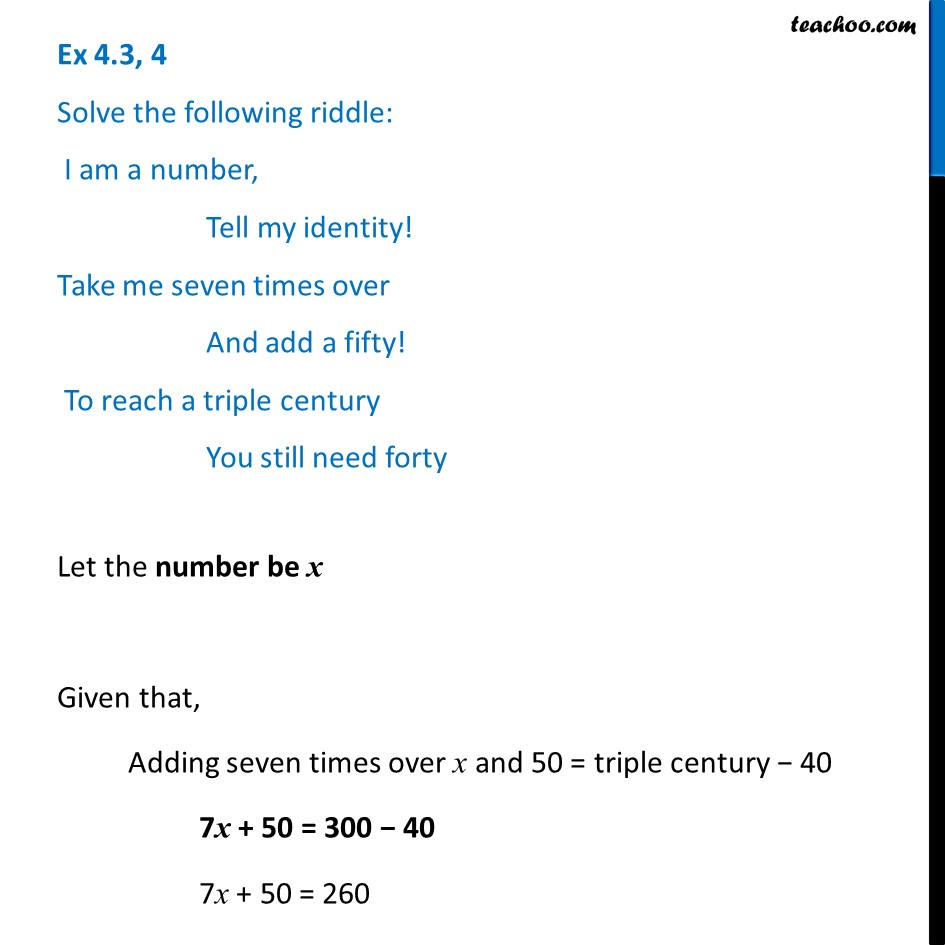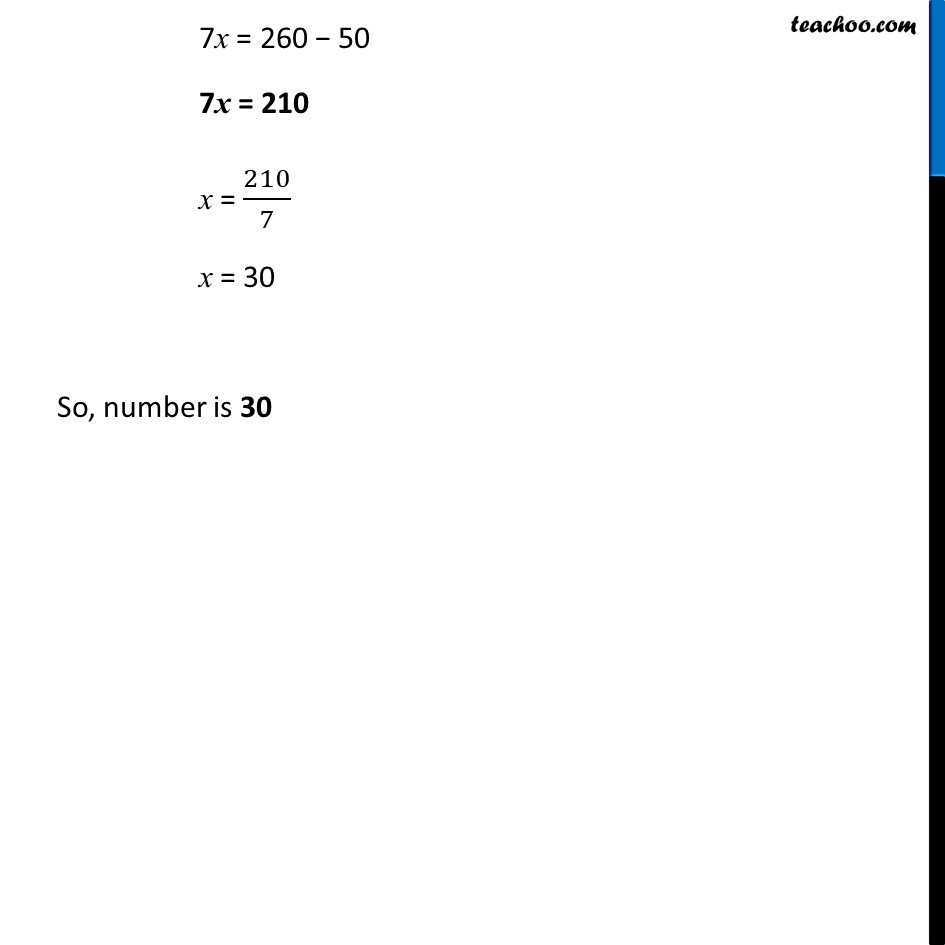Ex 4.3

Chapter 4 Class 7 Simple Equations
Serial order wiseLearn in your speed, with individual attention - Teachoo Maths 1-on-1 Class

### Transcript

Ex 4.3, 4 Solve the following riddle: I am a number, Tell my identity! Take me seven times over And add a fifty! To reach a triple century You still need fortyLet the number be x Given that, Adding seven times over x and 50 = triple century − 40 7x + 50 = 300 − 40 7x + 50 = 260 7x = 260 − 50 7x = 210 x = 210/7 x = 30 So, number is 30